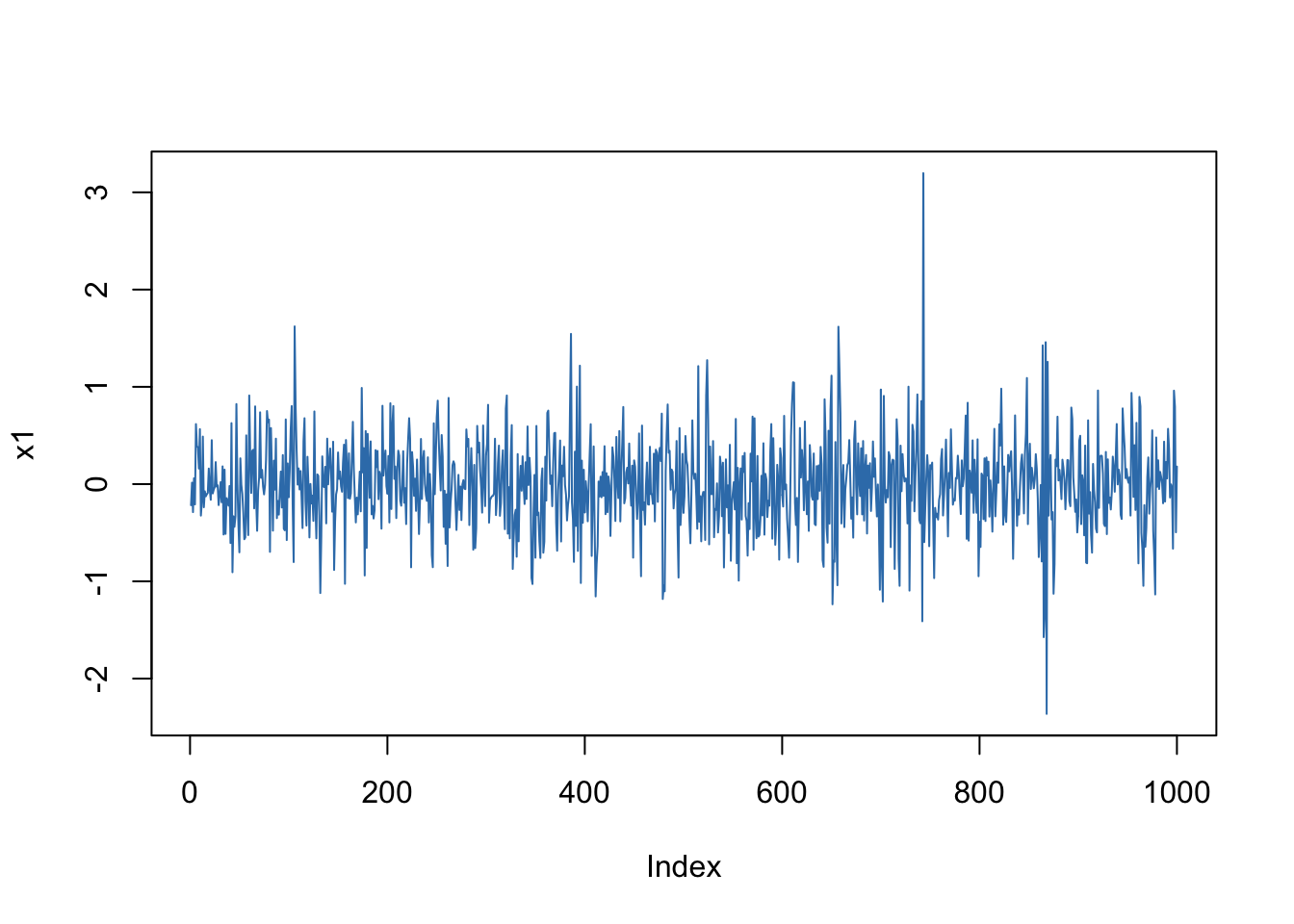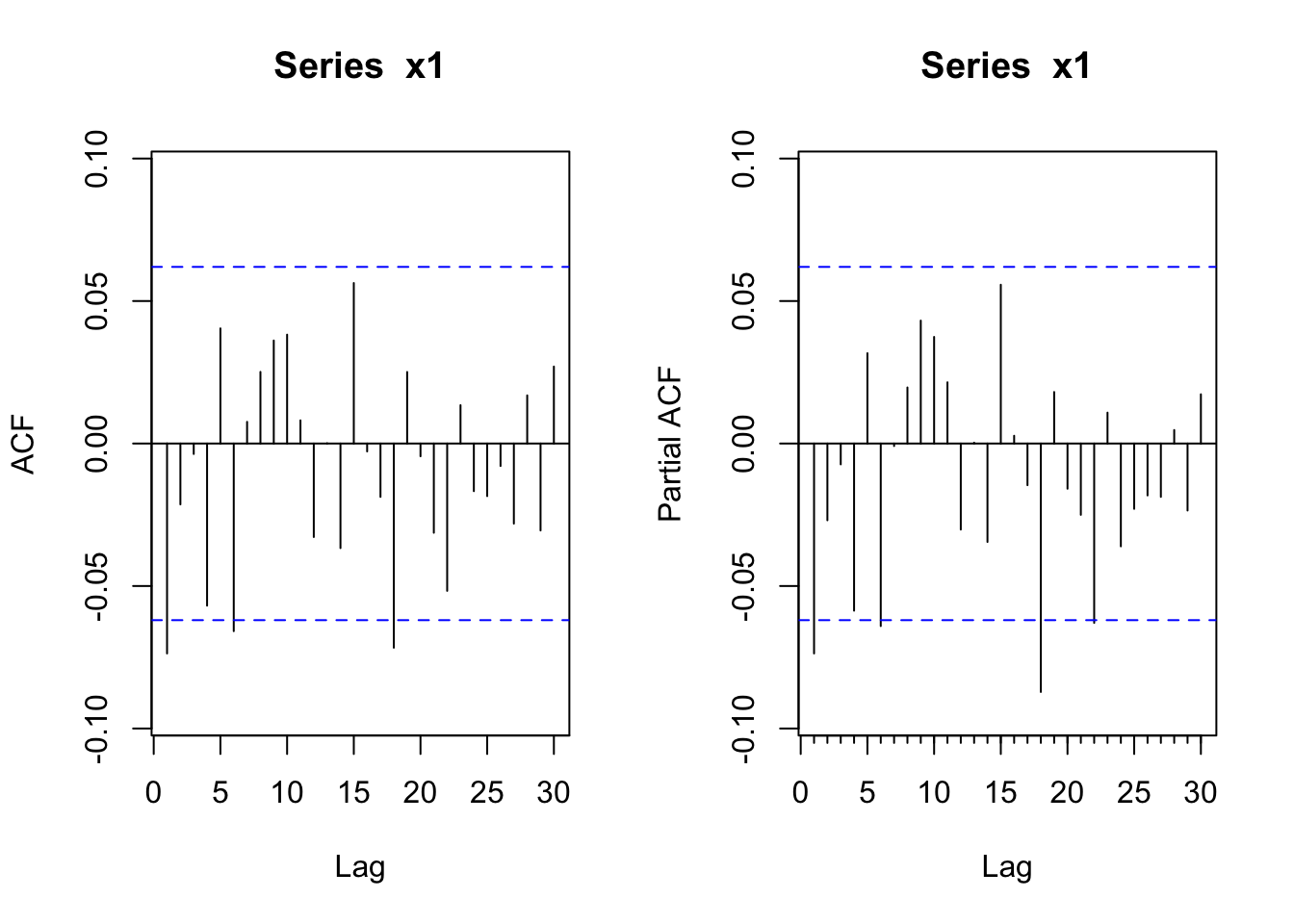## Preamble

``````library("forecast")
library("rugarch")``````
``Loading required package: methods``
``Loading required package: parallel``
``````
Attaching package: 'rugarch'``````
``````The following object is masked from 'package:stats':

sigma``````

## Simulating ARCH(1)

``````n <- 1100
omega <- 0.1
alpha <- 0.5

e <- rnorm(n)
x <- numeric(n)
x <- rnorm(1, sd = sqrt(omega/(1.0-alpha)))
for(i in 2:n) {
h <- omega + alpha * x[i-1]^2
x[i] <- e[i] * sqrt(h)
}
x1 <- x[101:1100] # throw away "burn-in"``````

Time Series Plot

``plot(x1, type="l", col=2)``ACF, PACF of Series

``````par(mfrow=c(1,2))
Acf(x1)
Pacf(x1)``````ACF, PACF of Squares

``````par(mfrow=c(1,2))
Acf(x1^2)
Pacf(x1^2)``````